The REG Procedure
 TEST Statement
<label:> TEST equation,<,...,equation> </ option> ;

The TEST statement tests hypotheses about the parameters estimated in the preceding MODEL statement. It has the same syntax as the RESTRICT statement except that it supports an option. Each equation specifies a linear hypothesis to be tested. The rows of the hypothesis are separated by commas.

Variable names must correspond to regressors, and each variable name represents the coefficient of the corresponding variable in the model. An optional label is useful to identify each test with a name. The keyword INTERCEPT can be used instead of a variable name to refer to the model’s intercept.

The REG procedure performs antest for the joint hypotheses specified in a single TEST statement. More than one TEST statement can accompany a MODEL statement. The numerator is the usual quadratic form of the estimates; the denominator is the mean squared error. If hypotheses can be represented by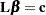then the numerator of thetest is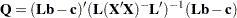divided by degrees of freedom, where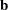is the estimate of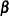. For example:

```model y=a1 a2 b1 b2;
aplus: test a1+a2=1;
b1:    test b1=0, b2=0;
b2:    test b1, b2;
```

The last two statements are equivalent; since no constant is specified, zero is assumed.

Note that, when the ACOV, HCC, or WHITE option is specified in the MODEL statement, tests are recomputed using the heteroscedasticity-consistent covariance matrix specified with the HCCMETHOD= option in the MODEL statement (see the section Testing for Heteroscedasticity).

One option can be specified in the TEST statement after a slash (/):

PRINT

displays intermediate calculations. This includes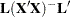bordered by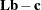, and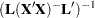bordered by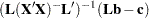.Previous Page | Next Page | Top of Page